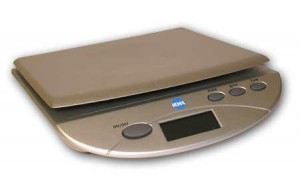# Grams to Ounces

We sometimes need to switch from metric tonon-metric units of measurement and vice versa. Sometimes they can be tricky even though the math isn’t that complicated. It really just involves a very simple calculation. However, the tricky part is knowing the conversion rate when you go from metric to non-metric units of measure. This is also the case when you go from grams to ounces.

### Which Ounce to Convert To

Another tricky part in converting grams to ounces is in the fact that there are two kinds of units that are known as ounces. Some might wonder if an ounce of gold will weigh as much as an ounce of plain rock. You might be surprised that an ounce of gold will be slightly heavier though we called both units of measure as ounces.

The fact is that we use a different unit of measure for precious metals, which we unfortunately also call an ounce. Well, this just adds to the confusion sometimes since everyone is very much used to converting grams to ounces using the regular conversion rate we use for liquids and other stuff.

Take note that when you’re converting precious metals, such as gold and silver, you will be using Troy ounces, which are slightly heavier. When converting regular material like fluids and other regular stuff you just bought from the store then you’ll be using what is known as Avoirdupois ounce, which has a slightly lower conversion rate compared to Troy ounces.

### Converting Grams to Ounces

Each type of ounce we have in real life has a different conversion rate. If you are converting a certain amount in grams to ounces, Avoirdupois ounce, then you will multiply the amount in grams by 0.0353. However, if you are converting from grams to Troy ounces then you will use a different conversion rate, which is 0.0322 since Troy ounces are slightly heavier than regular ounces.

### Conversion Examples

For instance, you used a calibrated scale to figure out the amount in grams of an everyday object or substance. Let’s say you determine it to be five grams. To convert this amount in grams to ounces use the conversion rate for Avoirdupois ounce, which will result to 0.1765 ounces.

Now, for example, your jeweler estimates the amount of gold in your necklace to be five grams. Multiplying this amount by the conversion rate for Troy ounces you will get 0.161. You can do the math on your own using the conversion rates for both types of ounces. Another option is to go online and use a search engine like Google for instance. Type amount of grams you want to convert, e.g. “5 grams”, and the unit of measure you want to convert to, e.g. “to Troy ounces”, then hit enter and voila.